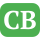CppBuzz.com

# C Conditional Statements - if else, if else if else

Conditional statements like if, else & else if are used in making a decision on certain conditions. These conditions are specified by a set of conditional statements having boolean expressions which are evaluated to a boolean true or false; that means conditional statements work true/false values. In C programming nested conditional statements are allowed.

## Boolean Operators

Before we dive into if else statements, we should know how Boolean operators works. These operators are called Boolean operators because the give true/false when we use them to test any condition.

 > Great than < Less than == Equality ! Not != Not equal >= Greater than or equal <= Less than or equal && And || Or

## The if statement

If if statement is true then we execute some set of statements. Or if statement is not true then execute some set of statements. To check in details lets look at an example -
```
#include<stdio.h>

int main()
{
int myno;
printf("\n Input any no :");
scanf("%d",&myno);
if ( myno == 102 )
printf("Myno is 102");
return 0;
}
```

In above program if user enters 102 then in if condition 102 == 120 which will be True; so if(true) will execute if block; since here if block has only one statement - "Myno is 102" is printed as output of the above program.

## The if else statements

```
#include<stdio.h>

int main()
{
int myno;
printf("\n Input any no :");
scanf("%d",&myno);
if ( myno == 102 )
printf("\n Myno is 102 Is equal");
else
printf("\n Myno is not 102");
return 0;
}
```

## The if else if else statements

```
#include<stdio.h>
int main()
{
int myno;
printf("\n Input any no :");
scanf("%d",&myno);
if ( myno == 102 )
printf("\n Myno is 102 Is equal");
else if(myno == 103)
printf("\n Myno is 103");
else
printf("\n Myno is niether 102 nor 103");
return 0;
}
```

## Nested if else statements

```
#include<stdio.h>
int main()
{
{
printf("\n Passed with Merits");